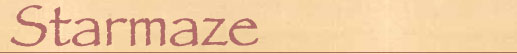If you spend any time wandering through the starmaze, you'll soon find yourself going in circles. Or, more accurately, going in squares: traveling in tight, four-step circuits I call "cycles." One of the first mysteries I encountered when exploring the starmaze was the odd fact that, with only four exceptions, the shortest path from any room back to itself always takes four steps.

This puzzled me until I understood that the starmaze is built on the edges of a nine-dimensional hypercube. Every move from one edge to the next in a cube requires a 90 degree turn, and no matter how many dimensions are involved, it always takes at least four moves to make a 360 degree turn and "come full circle." Those four 90 degree turns from a square, and there are many squares, or cycles, in the starmaze which all interlock in a complex "square dance".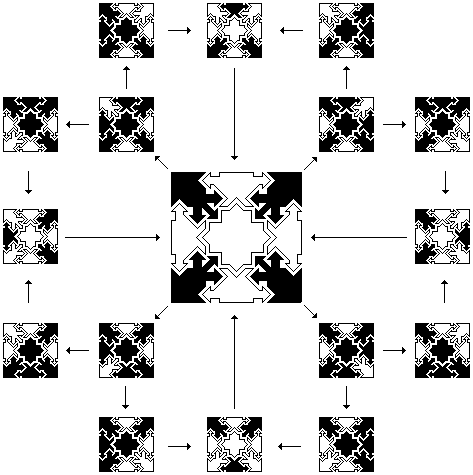The pattern at the center of the above diagram, pattern 170, participates in eight different cycles. For this reason it is called an "8-ring room." Some rooms have two cycles, some have four, some have six, and some have eight. There are only four "0-ring rooms" that do not lead into any cycles: the Pit, from which there is no escape, the Source, to which there is no return, the Starting Pattern and the Ending Pattern.

In addition to the 4 0-ring rooms, there are 112 2-ring rooms, 280 4-ring rooms, 112 6-ring rooms, and 4 8-ring rooms. When plotted on the starmap, they look like this: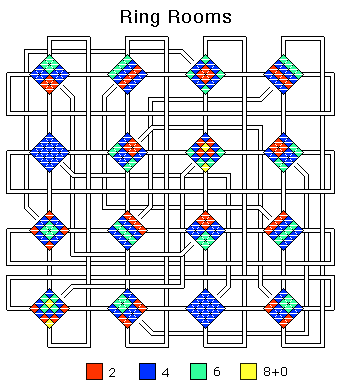If you study the map carefully, some of the properties of ring rooms become apparent. Notice that the two triangles comprising a room diamond are always the same color. Because the map places a room and its inverse in the same diamond, this tells us that the inverse of an n-Ring room is an n-Ring room. That is, the inverse of a 2-ring room is also a 2-ring room, the inverse of a 4-ring room is a 4-ring room, etc.

By following the center pipes, you can see that movement through the center cell from an n-ring room leads to an (8-n) ring room. That is, the center cell in a 2-ring room leads to a 6-ring room, the center cell in a 4-ring room leads to another 4-ring room, and the center cells of the four 8-ring rooms (170, 186, 325, and 341) lead to a 0-ring room.

Classifying these rooms is made easier using cycle diagrams. In a cycle diagram, open cells are indicated by filled diamonds. The state of the center cell is not shown, since the center cell is not involved in any cycles. Instead the center displays a sun if the pattern is a sun room, or a moon if the pattern is a moon room. Here are some cycle diagrams for 0-, 2-, 4-, 6-, and 8-ring rooms.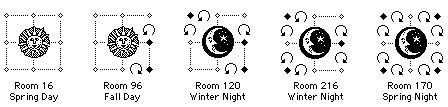The number and position of the cycles are indicated by the circular arrows. Notice that some cycles move clockwise and others move counter-clockwise; this is a property of seasons (discussed below). If you compare a room and its inverse, you will discover that the direction of each ring reverses in the inverted room.

It's quite easy to find the cycles emanating from a given pattern. Just look at the eight cells surrounding the center cell and find an open cell adjacent to a closed cell. For your first step choose the open cell; it will flip closed and, whatever else happens, the adjacent closed cell is guaranteed to flip open. For your second step, choose the previously closed cell. Again, both cells will flip. Now repeat the first two steps and you are back where you started.

There is always an even number of cycles emanating from any room. For example, patterns with a single open yin or yang cell (patterns of Form 1), will have an empty cell on either side. Depending on which empty cell you pick, you can travel through one of two different cycles. Even a pattern with a run of continuous open cells will have a closed cell on either end of the run. Both of these are examples of 2-ring rooms.

A pattern with two open cells separated by a closed cell (patterns of Form 3) are 4-ring rooms. Each of the two open cells emanates 2 cycles, one using the intervening closed cell and the other using one of the closed cells on either end. Replace the single intervening closed cell by a run of closed cells and the effect is unchanged: there are still a total of four cycles emanating from the pattern. 6-ring rooms, such as room 216 in the first diagram above, have three open cells each separated by intervening closed cells. And 8-ring rooms have four open non-adjacent cells. With this understanding, it's easy to see why the starting pattern is a 0-ring room: it has no open non-center cells at all.

Because each starmaze form includes both patterns and their inverses with and without the center cell open, all patterns of a given form emanate the same number of rings. The assignments are as follows:

 0-Ring Rooms: Form 20 2-Ring Rooms: Forms 1, 2, 6, and 13 4-Ring Rooms: Forms 3, 4, 5, 7, 8, 9, 10, 14, 15 and 18 6-Ring Rooms: Forms 11, 12, 16 and 17 8-Ring Rooms: Form 19# Seasons

As we've seen, each cycle in the starmaze follows a consistent sequence, cells A, B, A, B where A and B are two adjacent non-center cells, A initially open and B initially closed. If cell A is a yin cell, then this sequence is yin, yang, yin, yang. In the starmap, this translates as a journey through a macropipe to an adjacent microcosm, then a micropipe to an adjacent room, then a macropipe back to the original microcosm, then a micropipe back to the staring room. Similarly, if cell A is a yang cell, then the sequence is yang, yin, yang, yin - or micropipe, macropipe, micropipe, macropipe.

We can take advantage of this consistency to define the concept of seasons. Each room in the starmaze can be assigned a season (128 spring rooms, 128 summer rooms, 128 fall rooms, and 128 winter rooms) so that each cycle in the maze contains all four seasons. As you can see on the starmap, the room allocations are completely interspersed so that seasons change with every move through a micropipe or macropipe: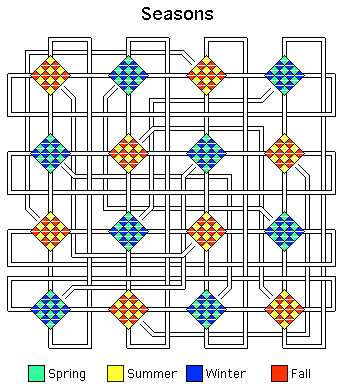The room allocations are made in much the same way as are sun and moon rooms. Just as a sun room is defined as a binary pattern with an even number of 0s, so each room in the starmaze can be assigned a unique season as follows:

 Spring: Even number of square yang cellsEven number of square yin cells Summer: Even number of square yang cellsOdd number of square yin cells Fall: Odd number of square yang cellsOdd number of square yin cells Winter: Odd number of square yang cellsEven number of square yin cells

By "square yang cell" I mean a binary coordinate of 1 in the position occupies by that cell (dimensions 1, 3, 7, or 9). This refers to the more recent system of drawing patterns in which circles indicate a binary coordinate of 0 and squares indicate a binary coordinate of 1.Consider, for example, pattern 400. As you can see, pattern 400 has one square yang cell (in the west) and one square yin cell (in the northwest). It's binary coordinates, using Lo Shu numbering, are 0,0,1,1,0,0,0,0,0. Since it has an odd number of yang square cells and an odd number of yin square cells, pattern 400 is assigned the Fall season.

This system of assigning seasons ensures that every single cycle in the starmaze includes a Spring room, a Summer room, a Fall room, and a Winter room. Moreover, these rooms will always occur in order (indicated by clockwise arrows in the cycle diagrams) or in exact reverse order (indicated by counter-clockwise arrows in the cycle diagrams). If you examine the above definition of the seasons you will see why: each movement will change the total oddness or evenness of either the yin cells or the yang cells, but not both at once. So Spring can change into either Summer or Winter, but never directly into Fall.

Again, let's take pattern 400 as an example. Pattern 400 is a 4-ring room, so we have four cycles to choose from, two emanating from the north yang cell, and two emanating from the southwest yin cell. Let's take the cycle formed by alternating between the north yang cell, cell 9, and the initially closed northeast yin cell, cell 2. Moving through cell 9 carries us to room 154 and changes cell 9 from a circle to a square. Because room 154 has 2 square yang cells and 1 square yin cell, it is a Summer room.We now choose cell 2, which carries us to room 456 and adds another square yin cell. With an even number of square yangs and yins, room 456 is a Spring room. Going back through cell 9 turns one of our square yangs back to a circle and carries us to room 194, a Winter room. The final move through cell 2 returns us to our starting point. We have traversed the four seasons, but we have moved backward in time from Fall to Summer to Spring to Winter and back to Fall, so that's why the circular arrow between cells 9 and 2 in room 400's cycle diagram is moving counter-clockwise.# Geometry

This system of assigning seasons can be made to work with any hypercube. So we can understand these seasonal cycles from a geometric point of view by considering the (relatively) simple case of the tesseract. One way of visualizing a tesseract is as an inner cube located "inside" an outer cube. Traditionally, yin is associated with even numbers and yang with odd numbers. So if we define the 1st (left/right) and 3rd (lower/upper) dimensions as yang dimensions and the 2nd (front/back) and 4th (outer/inner) as yin dimensions, and then apply the above definition of seasons, we can assign each room (corner) of the tesseract a season as follows:Here the origin, the room with coodinates (0,0,0,0), is located at the left, front, lower, outer corner. All its yin and yang coordinates are zero (even), so it is a Spring room. Moving along a yin dimension (from front to back or from outer to inner) will create an odd number of yin coordinates equal to one and result in a Summer room. Moving along a yang dimension, to (1,0,0,0) or (0,0,1,0) will result in a Winter room and would thus be considered a "backward" move.

Each of the six possible dimension pairs (1+2, 1+3, 1+4, 2+3, 2+4, and 3+4) form four faces in the tesseract (one for each of four possible states of the two dimensions excluded from the pair) for a total of 6 * 22 = 24. But only four of those six pairs (1+2, 1+4, 2+3, and 3+4) are comprised of both a yin and yang dimension, so only 4*4 or 16 faces form seasonal cycles.

For example, dimensions 1 and 2 form the face on the bottom of the outer cube, the bottom of the inner cube, the top of the inner cube, and the top of the outer cube; all four of these faces contain all four seasons. But faces formed by the pairs 1+3 or 2+4 form faces with only two seasons, like the frontward face of the outer cube; if you follow along the edges of that face you will shuttle back and forth between winter and spring without ever encountering a fall or summer corner.

Something analogous to this happens in the nine-dimensional starmaze. There are 9 choose 2 = (9*8)/2 = 36 possible dimension pairs in a 9-cube, each one generating 27 = 128 square faces for a total of 36 * 128 = 4608 square faces. But only 20 of those 36 dimension pairs are comprised of both a yin and yang dimension (each of the 4 yin dimensions can pair with any of the five yang dimensions for a total of 4 * 5 = 20). So of the 4608 faces in a 9-cube, only 20 * 128 = 2560 contain all four seasons.

This number, 2560, is also the maximum number of cycles it is possible to create in a 9 cube when you place directional arrows along each edge. To understand why this is, consider a single corner. To participate in a cycle, a corner has to have at least one edge coming into it and at least one edge going out. If it has, say, 1 edge going in and 8 going out, it could participate in, at most, 8 different cycles (depending how it's neighboring corners are configured). If it has 2 edges coming in and 7 going out, it could participate in as many as 2 * 7 = 14 different cycles. 3 and 6 would yield 18 possible cycles. The best possible case would be if it had either 4 edges coming in and 5 going out or 5 coming in and 4 going out; either way it could participate in up to 4 * 5 = 20 different cycles.

Now, if every single one of the 512 corners in a 9-cube could participate in 20 cycles, the total number of cycles in the 9-cube would be 512 * 20 / 4 = 2560. (We have to divide by 4 since otherwise we would be counting each cycle four times, one for each corner in the cycle.)

The same holds true in hypercubes of other dimensions. For even-numbered values of n, the best possible cycles/corner is n/2 * n/2; for odd-numbered values of n, best possible cycles/corner is (n-1)/2 * (n+1)/2. But this is exactly the same formula as the number of different ways to combine yin and yang dimensions. So the maximum number of cycles always works out to be equal to the number of 4-season cycles. And as the value of n increases, the maximum number of cycles in a n-cube approaches 50% as we can see in the following table:

Dimensions Total Squares Maximum Cycles Percentage
2 1 1 100.00%
3 6 4 66.67%
4 24 16 66.67%
5 80 48 60.00%
6 240 144 60.00%
7 672 384 57.14%
8 1792 1024 57.14%
9 4608 2560 55.56%
10 11520 6400 55.56%
20 49807360 26214400 52.63%
30 1.6769E+11 60397977600 51.72%
40 2.14405E+14 1.09951E+14 51.28%
50 3.44807E+17 1.75922E+17 51.02%
100 1.56872E+33 7.92282E+32 50.51%
1000 1.338E+306 6.6969E+305 50.05%

Is it always possible to choose directions for each edge in an n-cube to achieve the maximum possible number of cycles? The answer is yes - and this is where the concept of seasons proves useful. Once you assign a season to each of the 2n corners of an n-cube you can then use seasons to determine the direction of every edge by saying that Spring always leads to Summer, Summer to Fall, Fall to Winter, and Winter to Spring. The result would be an n-cube with the maximum number of possible cycles, each cycle containing 4 seasons all moving in a forward direction.

If you applied this technique to a 9-cube, for example, you would have a structure with no source and no sink. Every Spring and Fall corner would have five incoming edges and four outgoing edges; Every Summer and Winter corner would have four incoming edges and five outgoing edges. Dimension 5 would be just another yang dimension, not a special "center" dimension. One consequence of this is that, unlike the starmaze, moving through the center would not change the time of day (sun to moon) without affecting the season. So every Spring and Fall room would be a moon room and every Summer and Winter room a sun room. There would be no Spring days or Winter nights.

If we created patterns for every corner in the same we we do for the starmaze, half the patterns would have all the yang cells open (forming a cross) and the other half would have all the yin cells open in the four corners. Each move would jump from cross (sun) to corners (moon) or back again and the only difference between one cross pattern and another would be in its configuration of squares and circles representing the underlying binary address. Adjacent rooms would sometimes be three steps apart instead of just one because there would be no backward cycles; getting from a Spring room to a Winter room would always require three steps. It would still always take at least four moves to travel from a room back to itself, but there would always be exactly 20 different ways of doing this - so every room would be a 20-ring room.

This maximum cycle maze/puzzle is also a minimum coverage puzzle because it has only two patterns. As discussed in Puzzle Keys, the set of minimum coverage puzzles includes a set of maximum cycle puzzles. For order 9 puzzles, we expect to find the cross and corners puzzle just described, its inverse (which works the same way except that all the cycles run counter-clockwise), and 250 other variations which use different permutations of 4 or 5 open cells in each pattern). It's not yet clear whether or not all maximum cycle puzzles are minimum coverage puzzles.

The starmaze, then, is not the same as this "maximum cycle" maze, even though both are built on a 9 cube and both have exactly the same allocation of Spring, Summer, Fall, and Winter rooms in exactly the same locations. The difference is in the way the directional arrows are assigned to each of the 2304 edges of the 9-cube.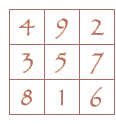In the starmaze, cycles only form along adjacent dimensions, not along any pair with both a yin and a yang. So instead of 20 different dimension pairs that can form cycles, there are only 8 (clockwise from the north: 9+2, 2+7, 7+6, 6+1, 1+8, 8+3, 3+4, and 4+9). The other difference is that dimension 5 is treated differently from the rest, so that any time you move across an edge in the 5th dimension, the arrangement of inbound vs. outbound edges always switches for all the yang dimensions and remains unchanged for all the yin dimensions.

These geometric differences give rise to the rules governing changes in the starmaze puzzle and vice-versa. In the starmaze, only adjacent dimensions can be involved in cycles because flipping an open cell in a starmaze pattern does not affect non-adjacent cells. If you flip an open cell and then flip another non-adjacent open cell you will not be able to take the third step in the cycle because the first cell will remain closed. Center cells are never involved in cycles for the same reason.# Counting Cycles

As a consequence of its special geometric properties, there are far fewer than the maximum number of possible cycles in the starmaze: 512 instead of 2506. These 512 cycles can be arranged into 8 groups based on the 8 possible adjacent non-center dimension pairs (9+2, 2+7, etc.). Each of these groups contains 64 variations which can be numbered in a consistent way to produce a unique cycle number for every cycle in the starmaze.

It may not be immediately obvious why there are only 64 variations in each group instead of 27 = 128. The reason is that 3 of the other 7 dimensions not involved in a dimensional pair are not independent of that pair.

Consider the first group, which is based on all cycles forming from dimensions 9 and 2. Dimensions 6, 1, 8, and 3 are all completely independent from the first group. Flipping one or more cells in any of these dimensions has no effect on either cell 9 or cell 2. And each of the 16 possible combinations of these four cells creates another group 1 variation.

The example we saw above was a group 1 cycle: 400 to 154 to 456 to 194 and back to 400. Flipping dimension 6 produces another group 1 cycle: 481 to 235 to 441 to 179 and back to 481.Flipping dimension 6 caused cells 5, 7, 1 and 6 to flip in every pattern of the second cycle. But since cells 9 and 2 were unaffected, we could still proceed by alternating between cells 9 and 2. Another valid variation would be formed if dimension 1 were flipped, or if both 1 and 6 were flipped, etc.

Dimensions 4, 7, and 5, however, are not completely independent of cells 9 and 2. Flipping any one of these three causes either cell 9 or cell 2 to flip open or closed, which leaves cells 9 and 2 in the same state (either open or closed) and thus makes it impossible to alternate between them. But if two of the three dimensions are flipped, the effect cancels out. As a result, 4 of the 8 possible combinations of these dimensions can still produce valid variations, as summarized in the following chart.

Cell 4
Flipped?
Cell 7
Flipped?
Cell 5
Flipped?
Cycle?
No No No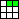Yes
Yes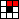No
Yes No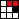No
YesYes
Yes No NoNo
YesYes
Yes No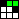Yes
Yes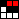No

Taken together, the 16 independent variations combined with the 4 valid dependent variations produces a total of 64 variations for each cycle group. So with 8 possible cycle groups the total number of unique cycles is 8 * 64 = 512.